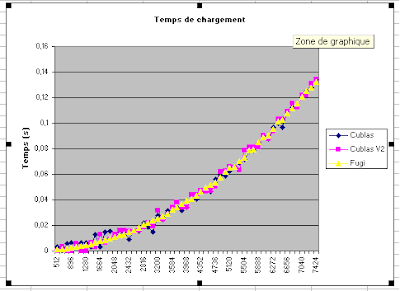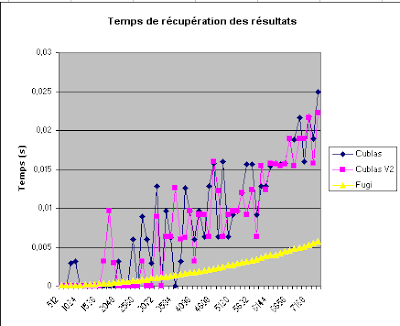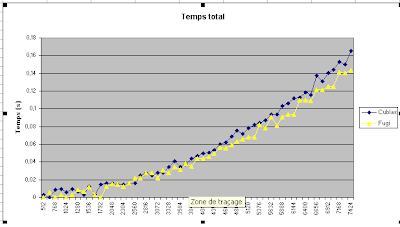# Bandwith differences between Cubblas and classical methods

Hi,

I work about the matrix product and for that I compare different method.

For the moment, I compare the classical Cubblas method with the Fujimoto’s algorithm

(Presented here).

The results between the two methods are at advantage for the Fuji algorithm, but after a comparison between the different step times (send data on the GPU, evaluated time and get the result). The difference (on two different GPU card) are only on the time read the result of the evaluation.

Time for send data on the GPUTotal TimeThe code for the cublas version is the following:

``````status = cublasAlloc(N*L, sizeof(d_A), (void**)&d_A);

status = cublasAlloc(K*N, sizeof(d_B), (void**)&d_B);

status = cublasAlloc(K*L, sizeof(d_C), (void**)&d_C);

status = cublasSetVector(N*L, sizeof(h_A), h_A, 1, d_A, 1);

status = cublasSetVector(K*N, sizeof(h_B), h_B, 1, d_B, 1);

status = cublasSetVector(K*L, sizeof(h_C), h_C, 1, d_C, 1);

float alpha = 1.0;

float beta = 0.0;

cublasSgemm('t', 'n', L, K, N, alpha, d_A, N, d_B, N, beta, d_C, N);

status = cublasGetError();

status = cublasGetVector(K*L, sizeof(h_C), d_C, 1, res.mat, 1);

status = cublasFree(d_A);

status = cublasFree(d_B);

status = cublasFree(d_C);
``````

I don’t understand why the Cubblas version loose it performance on the final step of the evaluation, what is different between the Cubblas final result and a classical “kernel” result explaining this difference?

Thanks a lot for your help

++ Beleys

Ps : I use a simple NVIDIA Quadro FX 570, (with CUBBLAS 2.0 version) and drivers : 6.14.11.7735

Calls to cublas operations are asynchroneous. Did you make sure you cudaSyncThreads before measuring the execution time? Otherwise your getMatrix call will take long because it waits for the Matrix-Multiplication to finish first.